# How can I apply calculation formulas to an input table using dropdown type?

•Rdiz
Asked on June 08, 2020 at 07:59 AM

I´m trying to set a simple add formula but using the dropdown type and setting a value depending on the response, can you help me?

I´m attaching my form, I want to have adding formula in every input table and as well as adding all the totals.

•shiro.ophal
Answered on June 08, 2020 at 08:40 AM

Hello!

You can do this with a simple calculation widget. It is a bit tedious to set up, but it will calculate everything automatically. In the calculation widget you've already set up, click "Add Field" then choose the dropdown question title (in this example "Resaca friendly. Elije tu preferida."):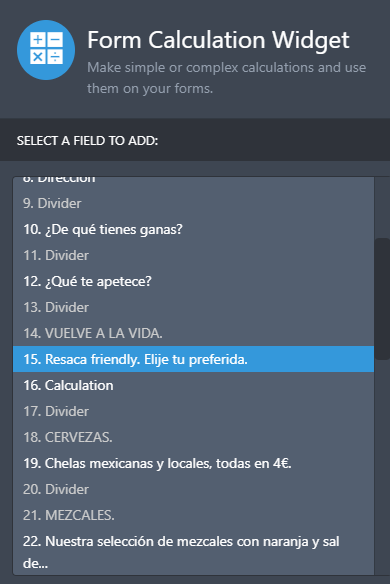Then choose one of the options to calculate a price on: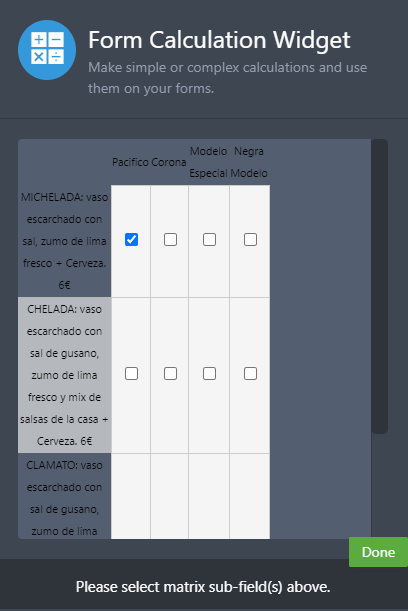Multiply this by the individual price of each item (in this example, 15):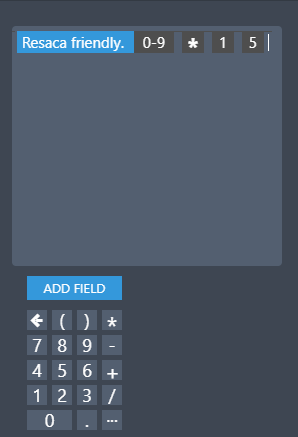You can then repeat these steps for each item, adding them together: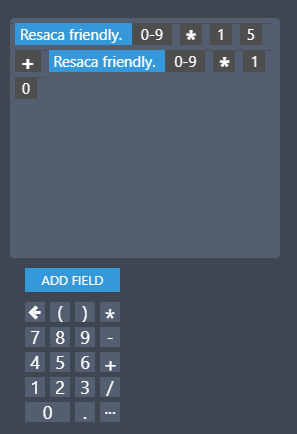This also works for the other dropdown menus below this one. In order to avoid having one calculation widget with a very long equation, I would recommend adding temporary ones which you can hide (or show to the customer as subtotals for each section):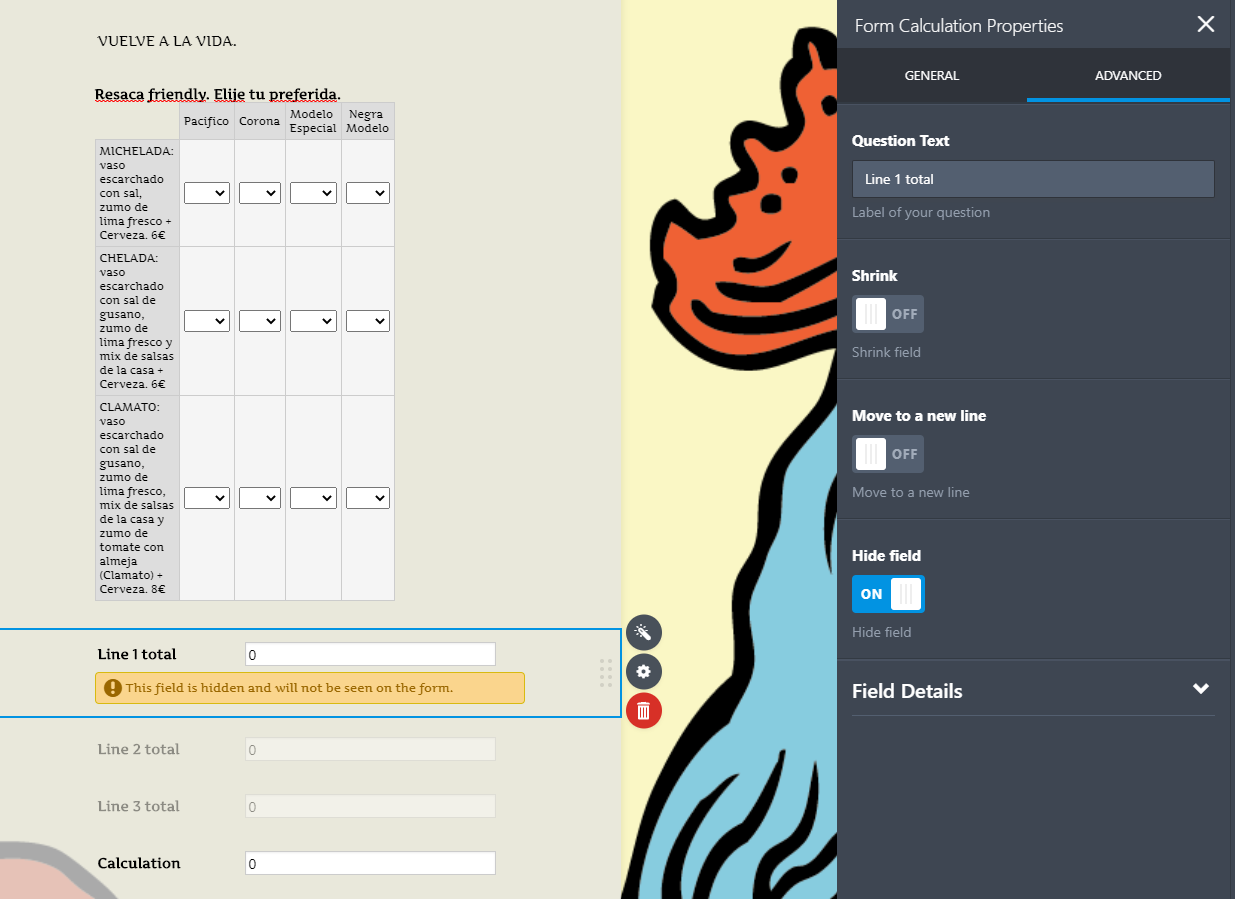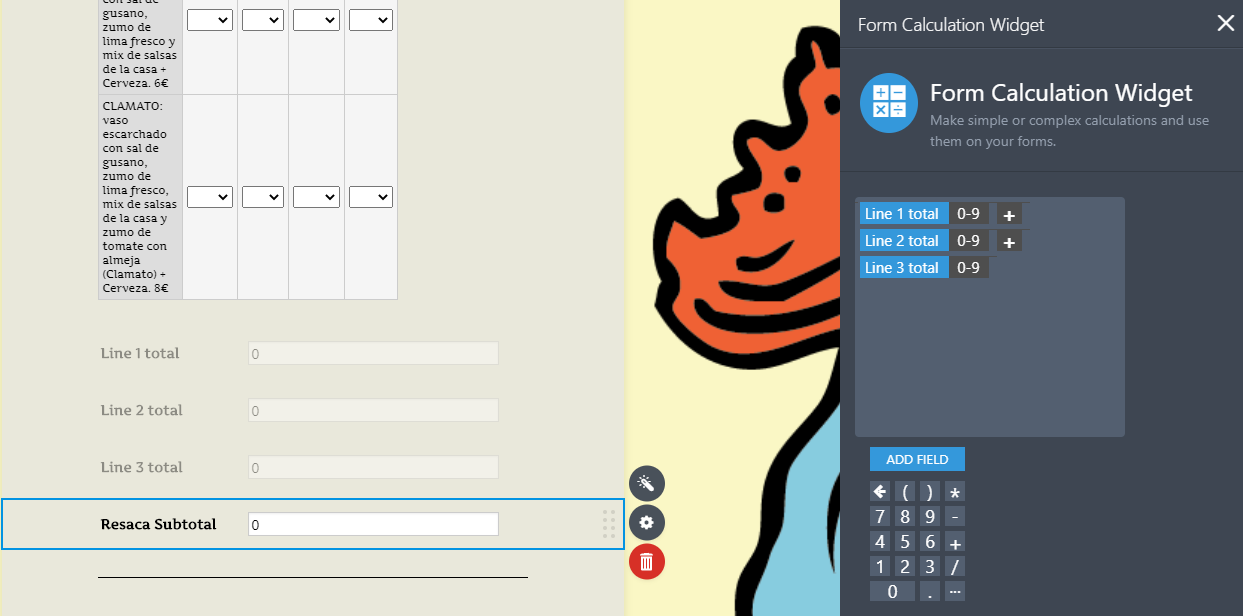And finally, add a final calculation form before payment method selection to sum all the intermediary ones: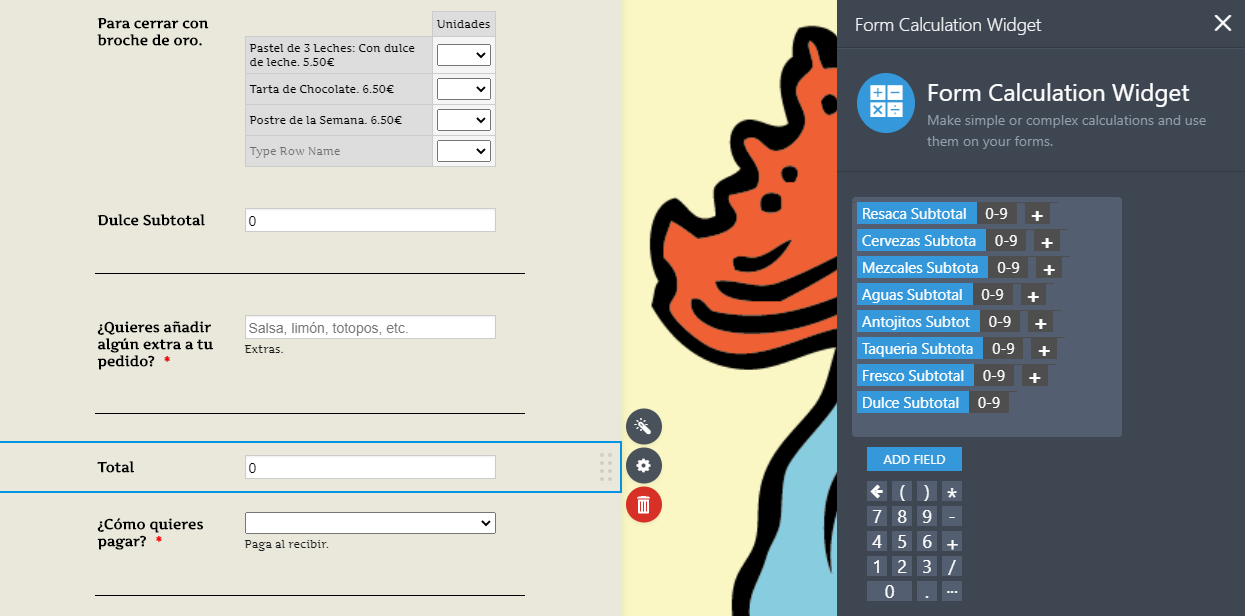•Richie_P
Answered on June 08, 2020 at 10:26 AM

Thank you shiro.ophal for the great instruction.

Hello Rdiz, if you have further questions, let us know.

•Rdiz
Answered on June 09, 2020 at 12:50 PM

Thank you very much!, All my questiones were answered and now I can finish my form.

Regards!